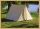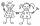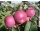# Equation - math word problems - page 51

1. Simple equation 5Solve equation with fractions: X × 3/8 = 1/2
2. BedsAt the summer camp, there are 41 chalets. Some rooms are 3-beds, some 4-beds. How many campers from 140 are living in 3-bed?
3. IntegerFind the integer whose distance on the numerical axis from number 1 is two times smaller as the distance from number 6.
4. ClothesDanka and Janka collect clothes. Danka had 9 more than Janka, so she gave her 7. Which now has more cloth and how many?
5. Cinema ticketsCinema sold 180 tickets this Thursday, which is 20%. Monday 14%, Tuesday 6%, Wednesday 9%, Friday 24%, Saturday 12%, and Sunday 15%. How many tickets were sold per week?
6. ThereThere are 32 trees of three species in the orchard. 25% cherries, three eighty pears, others plums. How many plums are in the orchard?
7. Reciprocal equation 2Solve this equation: x + 5/x - 6 = 4/11
8. A residentialA residential colony has a population of 5400 and 60 litres of water is required per person per day. For the effective utilization of rain water, they constructed a water reservoir measuring 48m × 27m × 25m to collect the rain water. For how many days, the
9. Company and employeesThere are 370 employees in the company - women are 15% less than men. How many men work in the company?
10. The priceThe price of the land increased by 17%. What was the original price of the land if it now costs 46800 €?
11. Seven timesWhich number seven times is just as higher as 27, how much is smaller than 29?
12. Father and sonWhen I was 11, my father was 35 years old. Today, my father has three times more years than me. How old is she?
13. 2 pipes2 pipes can fill a tank in 35 minutes. The larger pipe alone can fill the tank in 24 minutes less time than the smaller pipe. How long does each pipie take to fill the tank alone?
14. Average heightThe average height of all pupils is 162 cm. The class teacher's height is 178 cm. The average height of all (teacher and all pupils) is 163 cm. Calculate the number of pupils in the class.
15. Three peopleThree people start doing a work at a same time. The first worked only 2 hours. The second ended 3 hours before the end. On an individual basis, it would take the first time to do the work 10 hours, second 12 hours and 15 hours third. How many hours did it.
16. Dropped sheetsThree consecutive sheets dropped from the book. The sum of the numbers on the pages of the dropped sheets is 273. What number has the last page of the dropped sheets?
17. Number with onesThe first digit of the number is 1, if we move this digit to the end we get a 3 times higher number, which is the number?
18. 13 ticketsA short and long sightseeing tour is possible at the castle. Ticket for a short sightseeing circuit costs CZK 60, for a long touring circuit costs CZK 100. So far, 13 tickets have been sold for 1140 CZK. How much did you pay for tickets for a short tour?
19. The touristThe tourist traveled 190km in 5 hours. Part of the journey passed at 5 km/h. The rest he went by bus at a speed of 60 km/h. How long has a bus gone?
20. Two trainsThere were 159 freight wagons on the railway station creating 2 trains. One had 15 more wagons than the other. How many wagons did each train have?

Do you have an interesting mathematical word problem that you can't solve it? Enter it, and we can try to solve it.

To this e-mail address, we will reply solution; solved examples are also published here. Please enter the e-mail correctly and check whether you don't have a full mailbox.

Please do not submit problems from current active competitions such as Mathematical Olympiad, correspondence seminars etc...

Do you have a linear equation or system of equations and looking for its solution? Or do you have quadratic equation?